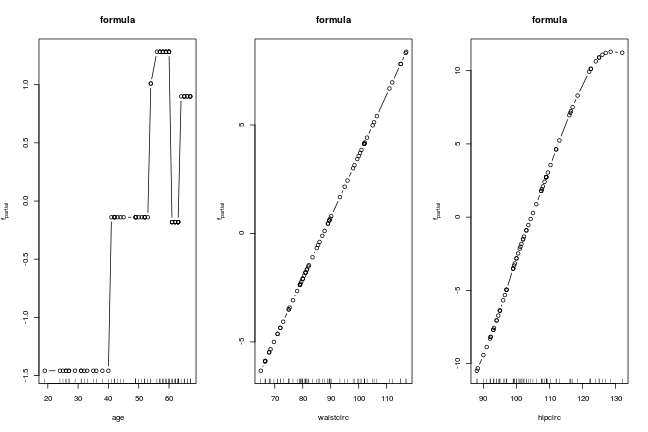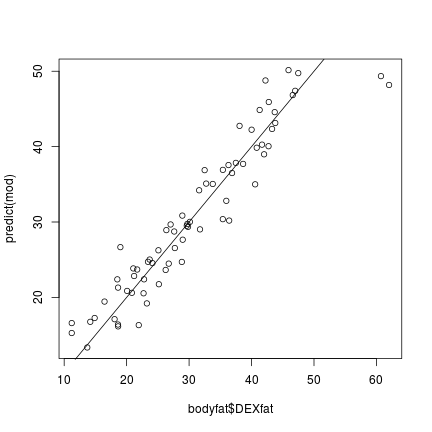First, install and fire-up R on your computer. Within R, one needs to install the mboost package by typing

install.packages("mboost")


and hitting the ENTER key. Once the package is installed, you can load it using

library("mboost")

## Loading required package: parallel

## Loading required package: stabs

## This is mboost 2.8-1. See 'package?mboost' and 'news(package  = "mboost")'
## for a complete list of changes.


Now all mboost functions are ready to be used, for example the mboost() function for fitting an additive regression model to the bodyfat data

data("bodyfat", package = "TH.data")

### formula interface: additive Gaussian model with
### a non-linear step-function in age', a linear function in waistcirc'
### and a smooth non-linear smooth function in hipcirc'
mod <- mboost(DEXfat ~ btree(age) + bols(waistcirc) + bbs(hipcirc),
data = bodyfat)


The model can be plotted

layout(matrix(1:3, nc = 3, byrow = TRUE))
plot(mod, ask = FALSE, main = "formula")or used for computing predictions

summary(predict(mod))

##        V1
##  Min.   :13.35
##  1st Qu.:22.61
##  Median :29.50
##  Mean   :30.78
##  3rd Qu.:38.41
##  Max.   :50.15


which can be compared to the actual response values:

plot(bodyfat\$DEXfat, predict(mod))
abline(a = 0, b = 1)
`